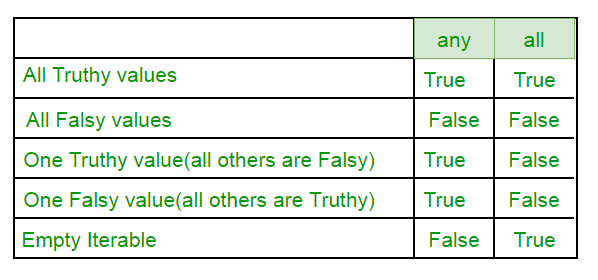GFG App
Open AppBrowser
Continue

# Any All in Python

Any and All are two built-in functions provided in python used for successive And/Or. Any Returns true if any of the items is True. It returns False if empty or all are false. Any can be thought of as a sequence of OR operations on the provided iterables. It short circuit the execution i.e. stop the execution as soon as the result is known.

Syntax:

`any(list of iterables) `

## Python

 `# Since all are false, false is returned` `print` `(``any``([``False``, ``False``, ``False``, ``False``]))`   `# Here the method will short-circuit at the` `# second item (True) and will return True.` `print` `(``any``([``False``, ``True``, ``False``, ``False``]))`   `# Here the method will short-circuit at the` `# first (True) and will return True.` `print` `(``any``([``True``, ``False``, ``False``, ``False``]))`

Output:

```False
True
True```

All Returns true if all of the items are True (or if the iterable is empty). All can be thought of as a sequence of AND operations on the provided iterables. It also short circuit the execution i.e. stop the execution as soon as the result is known.

Syntax :

`all(list of iterables) `

## Python

 `# Here all the iterables are True so all` `# will return True and the same will be printed` `print` `(``all``([``True``, ``True``, ``True``, ``True``]))`   `# Here the method will short-circuit at the ` `# first item (False) and will return False.` `print` `(``all``([``False``, ``True``, ``True``, ``False``]))`   `# This statement will return False, as no` `# True is found in the iterables` `print` `(``all``([``False``, ``False``, ``False``]))`

Output :

```True
False
False```

Practical Examples

## Python

 `# This code explains how can we ` `# use 'any' function on list ` `list1 ``=` `[]` `list2 ``=` `[]`   `# Index ranges from 1 to 10 to multiply` `for` `i ``in` `range``(``1``,``11``):` `    ``list1.append(``4``*``i) `   `# Index to access the list2 is from 0 to 9` `for` `i ``in` `range``(``0``,``10``):` `    ``list2.append(list1[i]``%``5``=``=``0``)`   `print``(``'See whether at least one number is divisible by 5 in list 1=>'``)` `print``(``any``(list2))`

Output:

```See whether at least one number is divisible by 5 in list 1=>
True```

## Python

 `# Illustration of 'all' function in python 3`   `# Take two lists ` `list1``=``[]` `list2``=``[]`   `# All numbers in list1 are in form: 4*i-3` `for` `i  ``in` `range``(``1``,``21``):` `    ``list1.append(``4``*``i``-``3``)`   `# list2 stores info of odd numbers in list1` `for` `i ``in` `range``(``0``,``20``):` `    ``list2.append(list1[i]``%``2``=``=``1``)`   `print``(``'See whether all numbers in list1 are odd =>'``)` `print``(``all``(list2))`

Output:

```See whether all numbers in list1 are odd =>
True```

Truth table: